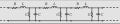# Thevenin equivalent of open ended transmission line

#### hoyyoth

Joined Mar 21, 2020
289
Dear Team,

I am going through the below link for understanding transmission line reflections.

The below figure is an equivalent circuit of an open-ended transmission line. For finding the voltage at V+ why the author used voltage division.

This is an open-ended transmission line so the voltage at V+ should be the open-circuit voltage Vg?

May I know where I went wrong?Regards

#### ericgibbs

Joined Jan 29, 2010
18,221
hi hoy,
The author is taking the internal resistance [Rg] of the Vsource into consideration in order to calculate the Vpd [V+] voltage step applied to the Transmission line.
E

Update:
As you are studying Transmission lines check out this video.

#### crutschow

Joined Mar 14, 2008
33,346
This is an open-ended transmission line so the voltage at V+ should be the open-circuit voltage Vg?
The steady state value will be the DC value of Vg, that's true.
But a step function will travel down the line with a value of Vg with an energy stored in the characteristic inductance and capacitance of the line.
When the step reaches the end, if it sees a resistive load matched to the lines characteristic impedance, this energy will be absorbed by the load.
If the line is open, then this energy will be reflected back down the line, adding a voltage on top of Vg.
For a lossless (ideal) line, this would double the voltage.

•hoyyoth

#### Irving

Joined Jan 30, 2016
3,544
This is an open-ended transmission line so the voltage at V+ should be the open-circuit voltage Vg?
That true for DC on an unbalanced short o/c, but a transmission line, with impedance Zc even with no load isn't an o/c, its a complex of series inductors/resistor and parallel capacitances, egSo there's a voltage divider effect with Rg. If the source had zero output impedance then you'd be correct.

Last edited:
•hoyyoth

#### ericgibbs

Joined Jan 29, 2010
18,221
My question is how can they apply voltage division to an open-ended line.
hi hoy,
Its not just an open ended line, its a distributed R/C/L network, which constitutes a load on the applied
Voltage step,

E
Watch the video .•hoyyoth

#### hoyyoth

Joined Mar 21, 2020
289
That true for DC on an unbalanced short o/c, but a transmission line, with impedance Zc even with no load isn't an o/c, its a complex of series inductors/resistor and parallel capacitances, egSo there's a voltage divider effect with Rg. If the source had zero output impedance then you'd be correct.
Hi Irving,

Thank you very much.
I got it.

Regards
Hari

#### ericgibbs

Joined Jan 29, 2010
18,221
hi hoy,
Not being critical of Irvings post. but if
If the source had zero output impedance then you'd be correct.

This Z=0 at the source would create other 'effects' on the line reflections calculations, as viewed from the Vsource perspective.
E

•hoyyoth and Irving

#### Irving

Joined Jan 30, 2016
3,544
hi hoy,
Not being critical of Irvings post. but if
If the source had zero output impedance then you'd be correct.

This Z=0 at the source would create other 'effects' on the line reflections calculations, as viewed from the Vsource perspective.
E
That may be so, but mathematically Rg =0 by definition means there is no voltage divider. And the thevenin equivalent is as viewed from the external perspective - in this case as viewed from Zc.

#### hoyyoth

Joined Mar 21, 2020
289
Dear Team,

One more question.
How the voltage at the open end of an open-ended transmission line becomes double the voltage of the rest of the line capacitance.

Regards
Hari

#### ericgibbs

Joined Jan 29, 2010
18,221
hi,
Its explained on the video at about 14 minutes .
E